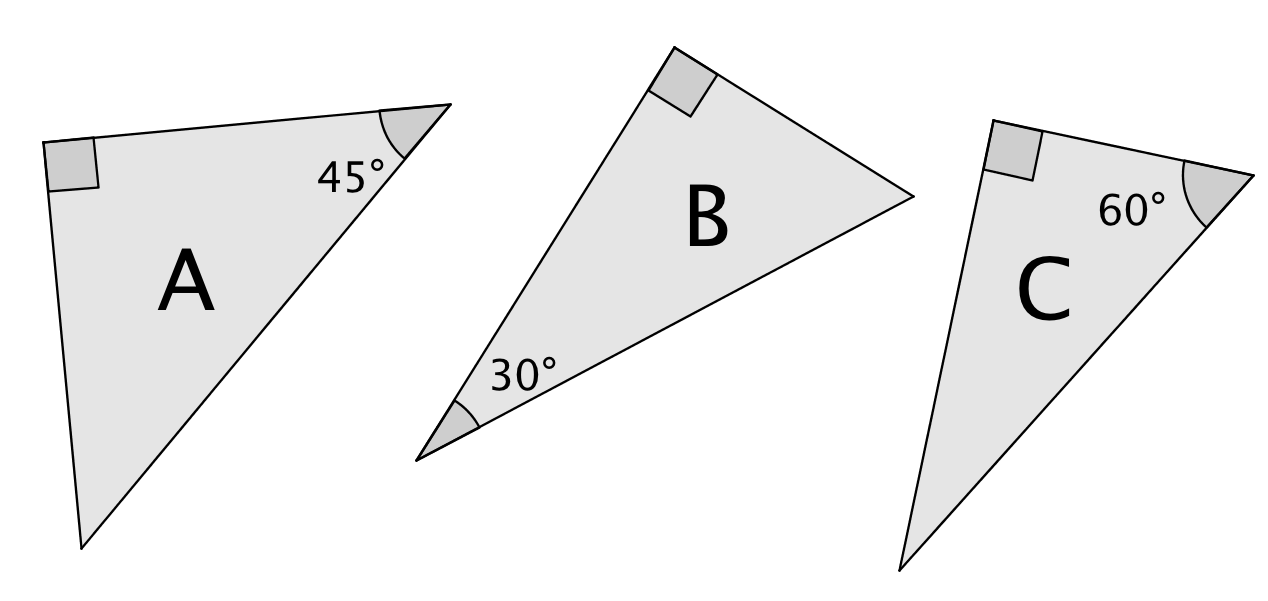# Right Triangles and Trigonometry

## Objective

Derive the relationship between sine and cosine of complementary angles in right triangles, and describe sine and cosine as angle measures approach 0°, 30°, 45°, 60°, and 90°.

## Common Core Standards

### Core Standards

?

• G.SRT.C.7 — Explain and use the relationship between the sine and cosine of complementary angles.

?

• G.CO.C.10

## Criteria for Success

?

1. Describe that the value of sine approaches 1 and the value of the cosine approaches 0 as an angle measure approaches 90°.
2. Describe that the value of sine approaches 0 and the value of the cosine approaches 1 as an angle measure approaches 0°.
3. Derive the relationship between sine and cosine of complementary angles in right triangles using the reference angles of 30°/60°, 45°/45°, 90°/0°.
4. Extend the relationship of sine and cosine of complementary angles to non-reference angles in right triangles. ​​​​​​

## Anchor Problems

?

### Problem 1

Below are three right triangles. Assume the value of the hypotenuse of each triangle is $1$.a) Using what you know about special right triangles, find the length of each side.
b) Fill in the chart describing the sine and cosine of each measure below.

 ${\mathrm{sin}(30^\circ)}$ ${\mathrm{sin}(45^\circ)}$ ${\mathrm{sin}(60^\circ)}$ ${\mathrm{cos}(30^\circ)}$ ${\mathrm{cos}(45^\circ)}$ ${\mathrm{cos}(60^\circ)}$

### Problem 2

Using the cosine and sine values from the table in Anchor Problem #1, identify trigonometric ratios that are the same. Then, write a conjecture about how the sine is related to the cosine of complementary angles.

## Problem Set

?

The following resources include problems and activities aligned to the objective of the lesson that can be used to create your own problem set.

FInd the value of ${\theta}$ that makes each statement true.
$\mathrm{sin}{\theta}=\mathrm{cos}32$
$\mathrm{cos}{\theta}=\mathrm{sin}({\theta}+20)$